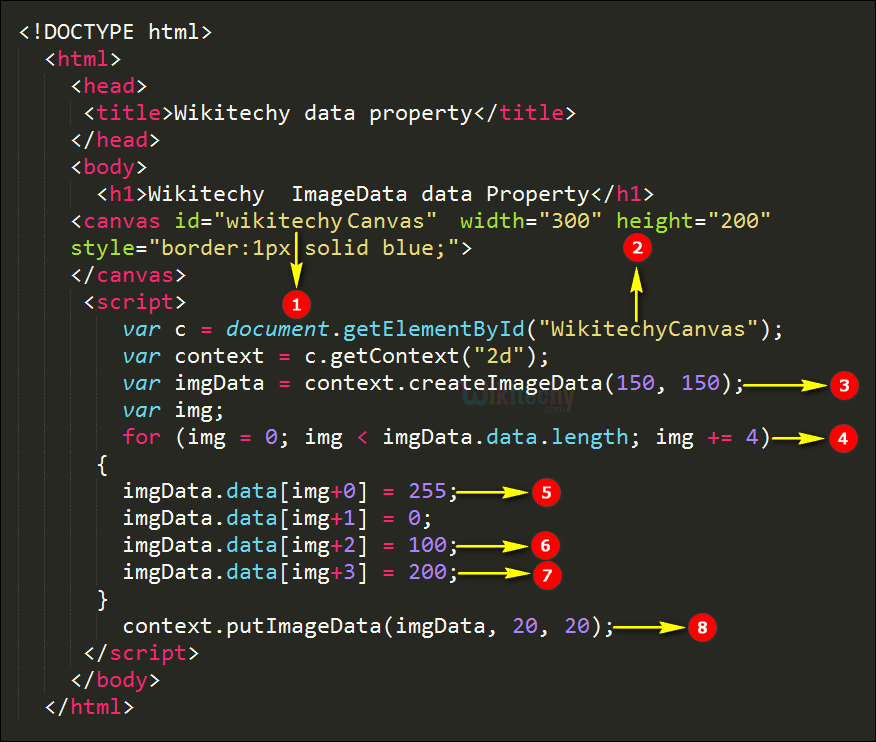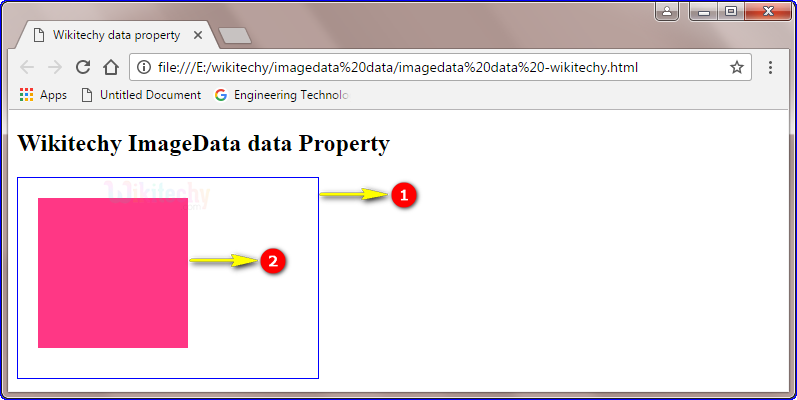# html tutorial - imageData data Property in HTML5 Canvas - html5 - html code - html formLearn html - html tutorial - Data property in html5 canvas - html examples - html programs

• The ImageData data is the Property of HTML canvas.
• The data property returns an object that contains image data of the specified ImageData object.
• Every pixel in an imageData object has four pieces of information, there are RGBA value.
• R- The red color (from 0 to 255).
• G- The green color (from 0 to 255).
• B-the blue color (from 0 to 255).
• A-the alpha channel (from 0 to 255)
• Where 0 s transparent and 255 is fully visible.
• The alpha/ color information is held in array and is stored in the data property of the imageData object.

## Code Explanation for imageData data Property in HTML5 canvas:1. ”wikitechyCanvas” is used to define the value id attribute for canvas element.
2. The getElementById(); method is used to get the element which has id as “wikitechyCanvas”.
3. The createImageData(); method creates a new, blank ImageData object(150,150).
4. for loop executes until the data.length.
5. The image color will be the combination of RGB color first 255 points the Red Color.
6. The value of Blue is 100, and the value of Green is 0.
7. The Alpha channel declared the value is 200
8. The
9. The putImageData() method puts the image data from a specified imageData object back onto the canvas.(imgData,20,20).

## Output for imageData data Property in HTML5 canvas:1. The canvas Rectangle with blue border.
2. The output shows pink color rectangle. Because, the imageData data property of RGBA values as Red is 255, Green is 0, Blue is 100 and Alpha value is 200 the value combination shows the pink color.

## Browser Support for imageData data Property in HTML5 canvas:Yes 9.0 Yes Yes Yes